The GAMPL Procedure

Model Evaluation Criteria

Given a fixed set of smoothing parameters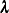in which each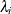controls the smoothness of each spline term, you can fit a generalized additive model by the penalized likelihood estimation. There are infinitely many sets of smoothing parameters. In order to search optimum models, some model evaluation criteria need to be defined to quantify the model goodness-of-fit. The GAMPL procedure uses the following model evaluation criteria:

• generalized cross validation (GCV),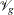(Craven and Wahba 1979)

• unbiased risk estimator (UBRE),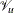(Craven and Wahba 1979)

• generalized approximate cross validation (GACV),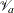(Xiang and Wahba 1996)

Consider the optimization problem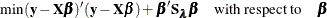The parameter estimate for
can be represented as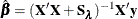And the smoothing matrix (also called the influence matrix or hat matrix) is thus represented as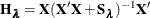With the defined smoothing matrix, you can form the model evaluation criteria as follows: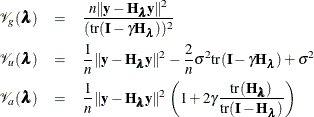In the equations,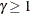(which corresponds to the GAMMA= suboption of the CRITERION= option) is the tuning parameter that is sometimes used to enforce smoother models.

The GAMPL procedure uses fitting algorithms that involve minimizing the model evaluation criterion with respect to unknown smoothing parameters.